# Chapter Notes - Measures of Dispersion Notes | Study Economics Class 11 - Commerce

## Commerce: Chapter Notes - Measures of Dispersion Notes | Study Economics Class 11 - Commerce

The document Chapter Notes - Measures of Dispersion Notes | Study Economics Class 11 - Commerce is a part of the Commerce Course Economics Class 11.
All you need of Commerce at this link: Commerce

Measures of Dispersion

1. Dispersion refers to the variation of the items around an average.

2. Objectives of Dispersion

a) To determine the reliability of an average.

b) To compare the variability of two or more series.

c) It serves the basis of other statistical measures such as correlation etc.

d) It serves the basis of statistical quality control.

Properties of good measure of Dispersion

a) It should be easy to understand.

b) Easy to calculate.

c) Rigidly defined

d) Based on all observations.

e) Should not be unduly affected by extreme values.

Measures of Dispersion may be either absolute measures or relative measure.

Absolute Measures of Dispersion are

a) Range

b) Quartile Deviation

c) Mean Deviation

d) Standard Deviation

Relative Measures of Dispersion are

a) Coefficient of Range

b) Coefficient of Quartile Deviation

c) Coefficient of Mean Deviation

d) Coefficient of Variation

Graphical method of dispersion

Lorenz Curve

Range

It is the difference between the largest and smallest value of distribution.

Computation of Range

Range = L – S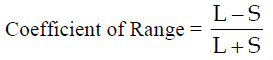Merits of Range

1. It is simple to understand and easy to calculate.

2. It is widely used in statistical quality control.

Demerits of Range

1. It is affected by extreme values in the series.

2. It cannot be calculated in case of open end series.

3. It is not based on all items.

Inter quartile range and quartile deviation

Inter quartile range is the difference between Upper Quartile (Q3) and Lower Quartile Q1.

Quartile deviation is half of inter quartile range.

Computation of Inter quartile range and quartile deviation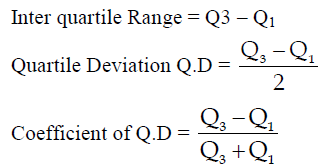Merits of Q.D

1. Easy to compute

2. Less affected by extreme values.

3. Can be computed in open ended series.

Demerits of Q.D

1. Not based on all observations

2. It is influenced by change in sample and suffers from instability.

Mean Deviation

Mean Deviation is defined as the arithmetic average of the absolute deviations [ignoring signs]

of various items from Mean or Median.

Computation of Mean Deviation

Individual Series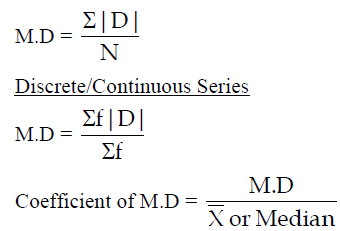Merits of Mean Deviation

1. Based on all observations.

2. It is less affected by extreme values.

3. Simple to understand and easy to calculate.

Demerits of Mean Deviation

1. It ignores ± signs in deviations.

2. It is difficult to compute when deviations comes in fractions.

Standard Deviation: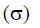It is defined as the root mean square deviation.

Features of Standard Deviation:

1. Value of its deviation is taken from Arithmetic Mean.

2. + and – signs of deviations taken from mean are not ignored.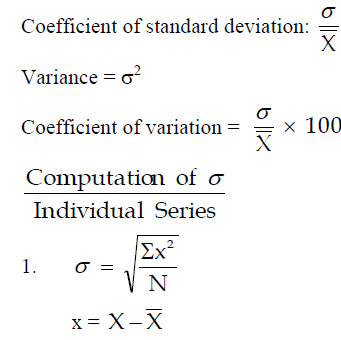Actual Mean Method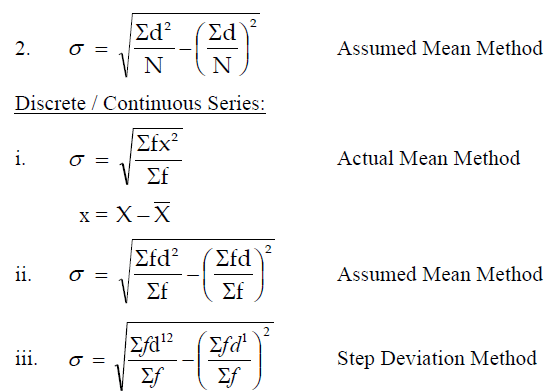Merits of Standard Deviation

i. Rigidly defined

ii. Based on all observations

iii. Takes Algebraic signs in consideration

iv. Amenable to further Algebraic treatment

Demerits

i. Difficult to understand and compute.

ii. Affected by extreme items.

Lorenz Curve

It is a graphical method of studying dispersion.

Lorenz curve is a cumulative percentage curve in which the percentage of frequency is combined

with percentage of other items such as profit, income etc.

The document Chapter Notes - Measures of Dispersion Notes | Study Economics Class 11 - Commerce is a part of the Commerce Course Economics Class 11.
All you need of Commerce at this link: CommerceUse Code STAYHOME200 and get INR 200 additional OFF

## Economics Class 11

201 videos|195 docs|64 tests

Track your progress, build streaks, highlight & save important lessons and more!

,

,

,

,

,

,

,

,

,

,

,

,

,

,

,

,

,

,

,

,

,

;